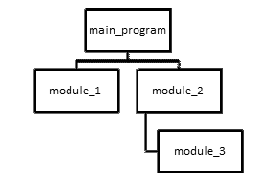In the previous article, we have discussed a little bit of Structure Theorem and array. Also the difference between procedural programming and object-oriented programming. In procedural programming, we divide general problem into more specific problems. In this article, Structure Theorem and array will be explained thoroughly, also introduction to modularization is given in this article.

STRUCTURE THEOREM

Structure Theorem is composed of sequential, selection, and repetition. Sequential means the program execution starts from top to bottom of the program. Selection is a representation of comparing two variables and take certain action(s). Repetition is repeating the same instruction(s) until certain condition is met. Below is the explanation and examples of selection and repetition.

• Selection

In pseudocode, there are two types of selection keywords. The first is IF…THEN…ELSE…ENDIF keywords to make simple selection. To have multiple selection options, rather than using nested ifs, we could use CASEOF…ENDCASE. In C, simple selection can be done using keywords if() and else(). Multiple selection options can also be done using keywords switch() and case.

• Repetition

In pseudocode, repetition has three categories, the first is leading, which in pseudocode is represented using keywords DOWHILE…ENDDO. This category examines the condition first, then if the condition is true, the following statements will be done. The second is trailing, which in pseudocode is represented using keywords REPEAT…UNTIL. Contrary to the first category, this category examines the condition at the tail of the repetition pseudocode. The last one is counted, which is represented using keyword DO…ENDDO. This category counts how many repetition has been done, and it stops after the repetition has been done for certain number/time.

ARRAY

Array, as explained in the previous article, is a data structure that consists data item of similar data type. In writing pseudocode, array can be written as array_name(array_size). In array, we can assign, process, search for, and display its values. These are the operations that can be done to an array.

In array, we know the term paired array. Paired array is not a two-dimensional array, but it is an array with equal size and each of its element corresponds to the element in the same index of another array. Both values are somehow bonded, but separate.

To search an array, two methods can be done: linear search and binary search. To do linear search, the elements in the array need not be sorted since linear search traverse each data exactly once. On the other hand, binary search needs to sort since binary search does comparison between the data searched and the middle data. Say we sort the array ascending, if the data searched is smaller than the middle data, the left side of the middle data will be used to search the data again; and vice versa.

MODULARIZATION

Modularization is needed to solve problem specifically, since it is the result of problem division. In pseudocode, the name of the module becomes the beginning of the pseudocode, ended with keyword END. The use of modularization is beneficial, because:

• it eases understanding,
• it is reusable,
• eliminates redundancy,
• and has good efficiency of maintenance.

To represent modularization and its structure, we can use hierarchy/structure chart. For example:To do modularization, we can do the following steps:

• define the problem (input, process, output),
• group activities into modules,
• create the hierarchy chart,
• create the pseudocode for the mainline of the program,
• develop pseudocode for the modules,
• do desk checking.

Sometimes, we need to pass parameter in developing modular programming. To do so, we can use the following syntax:

`module_name(param_1, param_2)`

However, if we use the above syntax for passing parameter in pseudocode, we cannot apply the same syntax to write array declaration.

To conclude, the structure theorem is very useful in both designing pseudocode and writing program. Also, the use of array and modularization in developing pseudocode is essential. Therefore, proper practice of developing pseudocode is needed in order to master programming design methods.